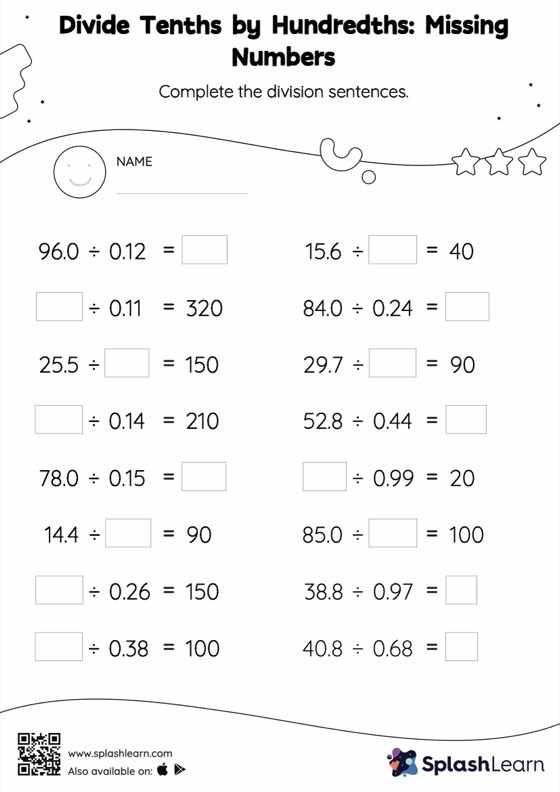# Divide Tenths by Hundredths without Remainder: Missing Numbers Worksheet

Home > Divide Tenths by Hundredths without Remainder: Missing NumbersCan your young mathematician divide tenths by hundredths without remainder? That's exactly what they do here. Students divide a whole number by a decimal without taking the decimal point into account, and then they place the decimal point at the appropriate place in the quotient depending on how many decimal digits there are in each number. This understanding helps them in finding the missing number in divide tenths by hundredths without remainder worksheet.